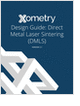MaterialsDesign CenterProcessesUnit ConversionFormulasMathematicsCalculatorsDiscussion ForumTrade PublicationsDirectory Service
Metal 3D Printing Design Guide

Direct Metal Laser Sintering (DMLS) 3D printing for parts with reduced cost and little waste.

Learn the best principles to negotiate the salary you deserve!

Essentials of Manufacturing

Information, coverage of important developments and expert commentary in manufacturing.

Salary Expectation

8 things to know about the interview question "What's your salary expectation"?

more free magazinesGlossary » Units » Power (Heat Flow) » Calorie (IT) Per SecondCalorie (IT) Per Second (cal (IT)/s) is a unit in the category of Power (Heat flow). It is also known as calories per second, calorie per second, cal/second, calorie/second. Calorie (IT) Per Second (cal (IT)/s) has a dimension of ML2T-3 where M is mass, L is length, and T is time. It can be converted to the corresponding standard SI unit W by multiplying its value by a factor of 4.18674.
Note that the seven base dimensions are M (Mass), L (Length), T (Time), Q (Temperature), N (Aamount of Substance), I (Electric Current), and J (Luminous Intensity).

Other units in the category of Power (Heat flow) include Abwatt (emu of Power) (aW), British Thermal Unit (IT) Per Hour (Btu (IT)/h), British Thermal Unit (IT) Per Minute (Btu (IT)/min), British Thermal Unit (IT) Per Second (Btu (IT)/s), British Thermal Unit (therm.) Per Hour (Btu (therm.)/h), British Thermal Unit (therm.) Per Minute (Btu (therm.)/min), British Thermal Unit (therm.) Per Second (Btu (therm.)/s), Calorie (IT) Per Hour (cal (IT)/h), Calorie (IT) Per Minute (cal (IT)/min), Calorie (therm.) Per Hour (cal (therm.)/h), Calorie (therm.) Per Minute (cal (therm.)/min), Calorie (therm.) Per Second (cal (therm.)/s), Cheval-Vapeur (horsepower) (cv, HP), Dyne-Centimeter Per Second (dyn-cm/s), Erg Per Second (erg/s), Foot-Pound Force Per Hour (ft-lbf/h), Foot-Pound Force Per Minute (ft-lbf/min), Foot-Pound Force Per Second (ft-lbf/s), Foot-Poundal Per Second (ft-pdl/s), Horsepower (550 Ft-Lbf/s) (HP), Horsepower (boiler) (HP), Horsepower (British) (BHP, hp), Horsepower (cheval-Vapeur) (cv, HP), Horsepower (electric) (HP), Horsepower (metric) (HP), Horsepower (water) (HP), Kilogram Force-Meter Per Second (kgf-m/s), Kilowatt (kW), Prony, Ton (refrigeration, UK) (CTR (UK)), Ton (refrigeration, US) (CTR (US)), Volt-Ampere (VA), Watt (W), Watt (int. Mean) (W), and Watt (int. US) (W).N/ARelated PagesMetal 3D Printing Design Guide

Direct Metal Laser Sintering (DMLS) 3D printing for parts with reduced cost and little waste.